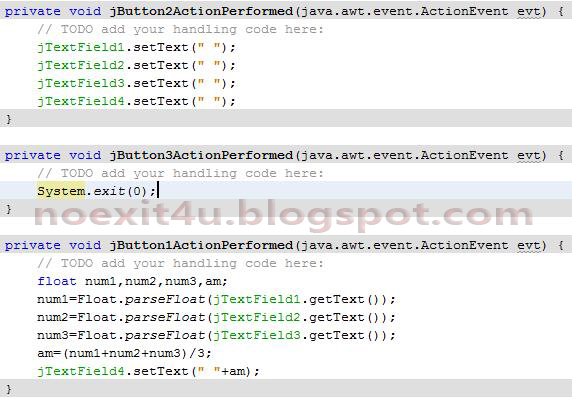## Thursday, 28 March 2013

### JAVA PROGRAM: TO CALCULATE ARITHMETIC MEAN OF THREE NUMBERS

CODING OF ARITHMETIC MEAN BUTTON(jButton1)
float num1,num2,num3,am;
num1=Float.parseFloat(jTextField1.getText());
num2=Float.parseFloat(jTextField2.getText());
num3=Float.parseFloat(jTextField3.getText());
am=(num1+num2+num3)/3;
jTextField4.setText(" "+am);

CODING OF CLEAR BUTTON(jButton2)
jTextField1.setText(" ");
jTextField2.setText(" ");
jTextField3.setText(" ");
jTextField4.setText(" ");

CODING OF EXIT BUTTON(jButton3)
System.exit(0);CODINGRUNNING

1.